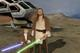• # Humpty Dumpty form

In Alice in Wonderland we are asked to return the volume and surface area of a spheroid. The assertion is that a sphere with a radius of 2 has a volume of 4.19 and a surface area of 12.57. The formula for a SPHERE (not spheroid) volume is 4/3(Pi)r^3 and for surface area is 4(Pi)r^2. Using these formulas, solving for r=2, the volume is correct at 4.19, but the surface area should be 50.27.

This is a substantial discrepancy. IS there something I am missing in the instructions?